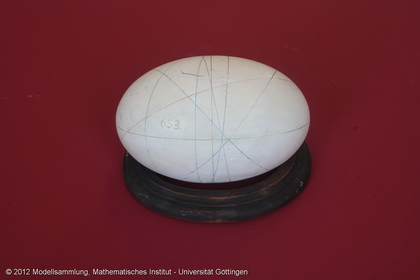Göttingen Collection of Mathematical Models and Instruments

# Extended rotational ellipsoid with 3 geodetic lines

### Model 653Constructor: Category: stud.math. K.Rohn, München A Ia 17

### Description

Extended rotational ellipsoid with 3 geodetic lines.

### Additions

This model depicts an spheroid obtained by rotating an ellipse of the equation $\frac{x^2}{4} + \frac{y^2}{4} + \frac{z^2}{9} = 1$ about its major axis.

“Die geodätischen Linien einer Fläche sind die Verallgemeinerung der Geraden in der Ebene” (Cohn-Vossen & Hilbert, 1996). “The geodesic lines of a surface are a generalization of straight lines in the plane” (Cohn-Vossen & Hilbert, 1996). In particular, they have the following three properties:

• Every sufficiently small section is the shortest connection of its end points on the surface.
• An infinitely small section gets pushed forward frontally (i.e. without leading in any other direction than forward).
• A geodesic line has the least possible curvature in any of its points, so in some sense it is the straightest one.

The model was published in the year 1877 as part of the series 1 by the company Martin Schilling. In 1911, it cost seven Goldmark. The original was manufactured at the mathematical institute of the royal technical university in Munich and was commissioned by Alexander Wilhelm von Brill.

Text written by: Jennifer Huhn and Theresa Lange

Translated by: Lea Renner

Showcase of this model is Case number 40

### References

Schilling, Martin(Hrg.): Catalog mathematischer Modelle, Leipzig(Verlag von Martin Schilling) 1911, 7.Auflage, No.213. p. 141.

Separataband M1 im Mathematischen Institut p. 25.

Hilbert, D.; Cohn-Vossen(1932). Anschauliche Geometrie, Springer-Verlag, Berlin. Online version